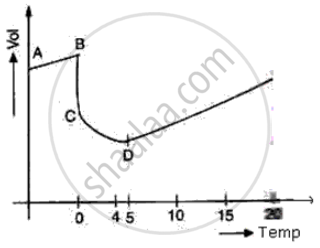# The diagram shows a sketch graph showing a change in volume of a given mass of ice at -5°C to water at 15°C. - Physics

Short Note

The diagram shows a sketch graph showing a change in volume of a given mass of ice at -5°C to water at 15°C.(i) Why does the temperature along BC remain constant?

(ii) When is the volume of water minimum?

(iii) At what temperature does water have maximum density? Explain.

#### Solution(i) At BC temperature is constant because at 0°C all of the heat given is used in the process of formation of water from ice and no part of the heat is used in changing the temperature of ice.

(ii) At point D i.e. at 4°C the volume of water is minimum

(iii) At point D i.e. at 4°C, the density of water is maximum due to anomalous expansion of water in the temperature range of 0°C and 4°C as volume decreases, the density of water increases

Concept: Expansion of Substances (Thermal Expansion) - Expansion of Solids
Is there an error in this question or solution?

#### APPEARS IN

Frank Class 9 Physics ICSE
Chapter 5 Heat
Thermal Expansion | Q 11 | Page 202